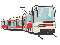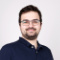# Nette\Database: Problem with newlines in where()

Notice: This thread is very old.Eda
Member | 216
+
0
-

Hi.

I have following code in presenter:

``````\$this->db->table('table')->where('
(id = ?) OR
(id = ?) OR
(id = ?)', '1', '2', '3');``````

It ends with warning: `Undefined offset: 1`.

It doesnt work properly on every db scheme I tryed. You can use this:

``````SET NAMES utf8;
SET foreign_key_checks = 0;
SET time_zone = 'SYSTEM';
SET sql_mode = 'NO_AUTO_VALUE_ON_ZERO';

DROP TABLE IF EXISTS `table`;
CREATE TABLE `table` (
`id` int(11) NOT NULL AUTO_INCREMENT,
PRIMARY KEY (`id`)
) ENGINE=InnoDB DEFAULT CHARSET=utf8 COLLATE=utf8_czech_ci;``````

I am using latest Nette 2.1-dev.

Note: workaround for this problem is to delete newlines in where, for example:

``````\$this->db->table('table')->where('
(id = ?) OR (id = ?) OR (id = ?)',
1, 2, 3);``````

Although there is a simple workaround, this problem is IMO unpleasant bug, which needs to be fixed.Bernard Williams
Member | 207
+
0
-

Small hint:

Hi,

better solution for this kind of query is:

``\$this->db->table('table')->where('id IN (?))', array(1, 2, 3));``

BernardEda
Member | 216
+
0
-

Hi. Yes, I know :-)
In original script I have something like that:

``````\$this->db->table('table')->where("
column1 > ? OR
(column2 = ? AND column3 < ?)
")``````

So theres no way to write it simplier.hrach
Member | 1819
+
0
-Eda
Member | 216
+
0
-

Thx for fix. …even with test! :-)

Works fine.hrach
Member | 1819
+
0
-

everything should be tested. I know the situation it's not ideal but I'm trying :))